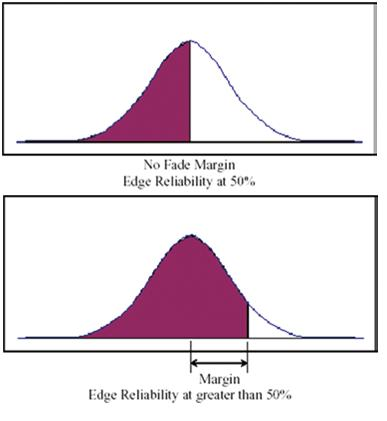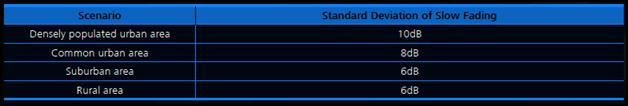# Slow Fading Margin in LTE with example of standard deviations in slow fading

Statistics repeatedly show that the median levels of received signals follow log-normal distribution with the time and location at a certain distance. Fading caused by location (mainly from obstruction) far exceeds fading caused by time. Therefore, the major concern for shadow fading is those caused by location changes.

To minimize the effect of shadow fading and ensure a certain edge coverage probability, certain allowances must be made. This is called the “slow fading margin”, or the “shadow fading margin”. Figure below shows the relationship between the slow fading margin and cell edge probability.The difference found in the slow fading is reflected by the standard deviation of slow fading. The standard deviation of slow fading shows the distribution of the radio signal strength at different test points at similar distances from the transmitter.

This spread of values approximate to the standard deviation of the signal strength at different test points from similar distances. The standard deviation of slow fading varies with the geological form.

The value ranges from 5 dB to 12 dB. In plain areas, such as rural areas and open areas, the standard deviation of slow fading is lower than that in suburban and urban areas. Standard deviations requirement in Dense Urban area with highly integrated building layout and deeper indoor coverage requirement is even higher than typical urban environment. Table below lists the typical standard deviations of slow fading in different geological locations.

Typical example of standard deviations in slow fadingThe slow fading margin can be obtained based on the cell edge coverage probability and standard deviation of slow fading.

The formula for calculating the edge coverage probability is as follows:

Edge coverage probability = 1 – Q (Slow fading margin /Standard deviation of slow fading)